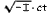Albert Einstein: Relativity
Appendix

## Minkowski's Four-Dimensional Space ("World") (supplementary to section 17)

We can characterise the Lorentz transformation still more simply if we introduce the imaginaryin place of t, as time-variable. If, in accordance with this, we insert

x1 = x
x2 = y
x3 = z
x4 =and similarly for the accented system K1, then the condition which is identically satisfied by the transformation can be expressed thus :

x1'2 + x2'2 + x3'2 + x4'2 = x12 + x22 + x32 + x42     (12).

That is, by the afore-mentioned choice of " coordinates," (11a) [see the end of Appendix II] is transformed into this equation.

We see from (12) that the imaginary time co-ordinate x4, enters into the condition of transformation in exactly the same way as the space co-ordinates x1, x2, x3. It is due to this fact that, according to the theory of relativity, the " time "x4, enters into natural laws in the same form as the space co ordinates x1, x2, x3.

A four-dimensional continuum described by the "co-ordinates" x1, x2, x3, x4, was called "world" by Minkowski, who also termed a point-event a " world-point." From a "happening" in three-dimensional space, physics becomes, as it were, an " existence " in the four-dimensional " world."

This four-dimensional " world " bears a close similarity to the three-dimensional " space " of (Euclidean) analytical geometry. If we introduce into the latter a new Cartesian co-ordinate system (x'1, x'2, x'3) with the same origin, then x'1, x'2, x'3, are linear homogeneous functions of x1, x2, x3 which identically satisfy the equation

x'12 + x'22 + x'32 = x12 + x22 + x32

The analogy with (12) is a complete one. We can regard Minkowski's " world " in a formal manner as a four-dimensional Euclidean space (with an imaginary time coordinate) ; the Lorentz transformation corresponds to a " rotation " of the co-ordinate system in the fourdimensional " world."